Time: 3 Hours                                                                                                     Max. Marks: 100

NOTE: There are 9 Questions in all.

·      Question 1 is compulsory and carries 20 marks. Answer to Q. 1. must be written in the space provided for it in the answer book supplied and nowhere else.

·      Out of the remaining EIGHT Questions answer any FIVE Questions. Each question carries 16 marks.

·      Any required data not explicitly given, may be suitably assumed and stated.

Q.1       Choose the correct or best alternative in the following:                                         (2x10)

a.       UJT is generally used for

(A)  Controlling the power.                  (B)  Triggering a triac.

(C)    Triggering an SCR.                      (D) Triggering a Diac.

b.      A single-phase full wave half controlled bridge rectifier uses

(A)    2 SCR’s.                                      (B)  4 SCR’s.

(C)  6 SCR’.                                       (D) 1 SCR.

c.   According to their connections, inverters are classified as

(A)    Series inverters.                            (B)  Parallel inverters.

(C)  Bridge inverters.                           (D)  All the types as above.

d.   In resistance welding the heat produced is proportional to the

(A)  current                                         (B)  square of the current

(C) voltage                                          (D) square of the voltage

e.   In a single-phase full wave controlled rectifier using centre tap transformer, the voltage across each half secondary is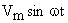.  The peak inverse voltage is

(A)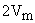.                                         (B)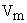.

(C)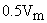.                                      (D)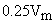.

(A)     Remains constant.

(B)     Varies between maximum and minimum values.

(C)  May remain constant or vary.

(D)  Is constant if R is constant.

g.   A cycloconverter can be

(A)     Step down.                                  (B)  Step up.

(C)  Step down or Step up.                 (D)  Neither of above.

h.   In resistance welding the heat produced is proportional to the

(A)     Current.                                       (B)  Square of the current.

(C)  Voltage.                                       (D)  Square of the voltage.

i.    Induction heating requires

(A)    AC input.                                     (B) High frequency AC input.

(C) DC input.                                      (D) No input.

j.    Inflammable articles like plastic and wooden products etc can be safely heated by using ___________ heating.

(A)   Eddy-current                                (B) Dielectric.

(C) Induction.                                      (D) Resistance.

Answer any FIVE Questions out of EIGHT Questions.

Each question carries 16 marks.

Q.2     a.   Explain the two transistor analogy of an SCR with suitable circuit diagram.  How does the gate function as the controlling element of the SCR?      (5+3)

b.   Explain resistance triggering and R-C triggering circuit of an SCR.                   (4+4)

Q.3     a.   Explain the principle of operation and application of a single-phase cyclo  converter.                       (8)

b.   A fully controlled 3-phase bridge rectifier circuit is fed by 400 V, 3-phase, 50 Hz supply.  The average load current is 150A and the load is highly inductive.  The firing angle is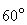.  Find:

(i)                  output power

(ii)                average rms and peak current through the thyristor

(iii)               peak inverse voltage.                                                                  (8)

Q.4     a.   Explain the different turn off circuits of an inverter.                                               (8)

b.   Give the different types of classification of inverters.  Also give the applications of series and parallel inverters.                                             (4+4)

Q.5     a.   Explain the principle of operation of chopper.  Give a few applications of choppers.            (6+2)

b.   Explain, how Jones chopper is different from Morgan’s chopper with circuit diagrams.                    (8)

Q.6     a.   Explain the process of dielectric heating and also give a few applications of the same.                      (5+3)

b.   Explain the basic circuit of resistance welding & give a few applications of resistance welding.                                                                    (5+3)

Q.7     a.   Explain the circuit of a single-phase half wave converter with R – load and without free wheeling diode.  Also draw the waveforms.                           (4+2)

b.   Explain voltage and current ratings of an SCR.                                                  (10)

Q.8     a.   Explain the principle of induction heating giving a few applications.                  (6+2)

b.   Why, is induction heating more advantageous? Explain, thermal loss in dielectric heating.                 (8)

Q.9           Write short notes on:-

(i)                  Turn ON methods of an SCR. (10)

(ii)                D.C. motor speed control using controlled rectifier.                                 (6)